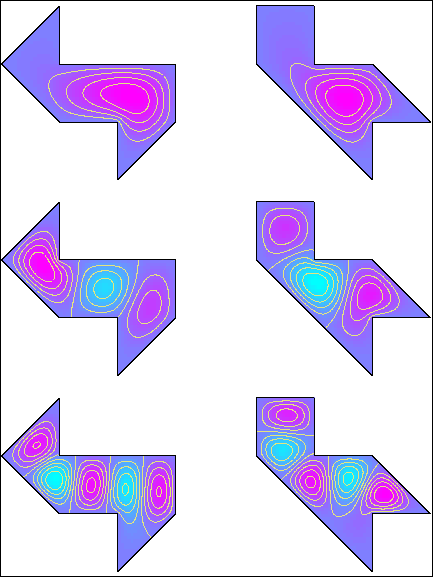Course Title: Partial Differential Equations MATH 6420 - 1 Andrejs Treibergs http://www.math.utah.edu/~treiberg/M6420.html M, W, F, 8:35-9:25 AM in LCB 323 10:40-11:30 M, W, F, in JWB 224 (tent.) treiberg@math.utah.edu Math 6410 or consent of instructor. Robert C. McOwen, Partial Differential Equations: Methods and Applications, Second Edition, Prentice Hall, Pearson Education, Inc., Upper Saddle River, NJ. 2003.This is the second semester in a year long graduate course in ordinary and partial differential equations. In this course, along with Math 6410, we shall try to cover the syllabus for the qualifying exam in differential equations. Although some mathematical sophistication is required to take the course, we shall provide any background materials needed by the class.

We shall follow McOwen's text covering first order equations, distributions, wave-heat-Laplace equations, L2-theory, energy methods, Sobolev spaces, regularity theory. Topics include (depending on time):

• Introduction to PDE: applications, variational problems.
• First Order Equations: method of characteristics, conservation laws, weak solutions.
• Principles of Higher Order Equations: distributions, Cauchy Kovalevsky Theorem, classification.
• Wave Equation: spherical means, energy methods.
• Laplace's equation: fundamental solution, perron's method, eigenvalues.
• Heat Equation: heat kernel, maximum principle, smoothness.
• Functional Analysis: weak solution of Poisson's Equation, Sobolev Inequality, Sobolev Embedding Theorem.
• Differential Calculus Methods: existence of a minimizer, Mountain Pass Lemma.
• Linear Elliptic theory: Sturm-Liouville Theory, Lp and Hölder estimates, regularity of weak solutions, elliptic maximum principle.

Last updated: 01 / 03 / 03